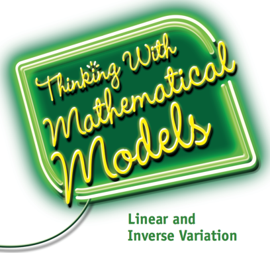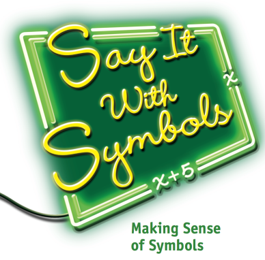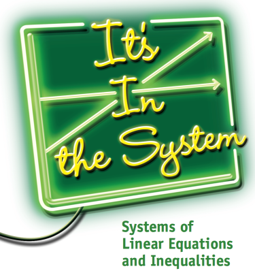Toggle Accessibility Tools

##Thinking With Mathematical Models

### Linear and Nonlinear Relationships: Recognize and model patterns in bivariate data.

• Represent data patterns using graphs, tables, word descriptions, and algebraic expressions
• Investigate the nature of linear functions in contexts
• Write linear functions from verbal, numerical, or graphical information
• Analyze and solve linear equations
• Model situations with inequalities expressed as "at most" and "at least" situations
• Investigate the nature of inverse variation in contexts
• Compare inverse variation relationships with linear relationships

### Data Analysis: Measure variation in data and strength of association in bivariate data.

• Use data to make predictions
• Fit a line to data that show a linear trend and measure closeness of fit
• Analyze scatter plots of bivariate data to determine the strength of the linear association between the two variables
• Use correlation coefficients informally to describe the strength of the linear association illustrated by scatter plots
• Use standard deviation to measure variability in univariate distributions
• Distinguish between categorical and numerical variables
• Use two-way tables and analysis of cell frequencies and relative frequencies to decide whether two variables are related

##Looking for Pythagoras

### Pythagorean Theorem: Understand and apply the Pythagorean Theorem.

• Develop strategies for finding the distance between two points on a coordinate grid
• Explain a proof of the Pythagorean Theorem
• Use the Pythagorean Theorem and its converse to solve a variety of problems
• Use the Pythagorean Theorem to find the equation of a circle with its center located at the origin

### Real Numbers: Understand that the set of real numbers consists of rational and irrational numbers.

• Interpret square roots and cube roots of numbers by making use of their related geometric representations
• Relate the area of a square to the side length of the square
• Estimate the values of square roots
• Estimate the values of cube roots
• Relate the volume of a cube to the edge length of the cube
• Compare numbers that can be represented as fractions (rational numbers) to numbers that cannot be represented as fractions (irrational numbers) and recognize that the set of real numbers consists of rational and irrational numbers
• Represent rational numbers as fractions and as terminating decimals or repeating decimals
• Recognize that irrational numbers cannot be represented as fractions and are nonterminating, nonrepeating decimals
• Recognize that the square root of a whole number that is not a square is irrational
• Locate irrational numbers on a number line
• Use and understand properties of rational and irrational numbers

##Growing, Growing, Growing

### Exponential Functions: Explore problem situations in which two or more variables have an exponential relationship to each other.

• Identify situations that can be modeled with an exponential function
• Identify the pattern of change (growth/decay factor) between two variables that represent an exponential function in a situation, table, graph, or equation
• Represent an exponential function with a table, graph, or equation
• Make connections among the patterns of change in a table, graph, and equation of an exponential function
• Compare the growth/decay rate and growth/decay factor for an exponential function and recognize the role each plays in an exponential situation
• Identify the growth/decay factor and initial value in problem situations, tables, graphs, and equations that represent exponential functions
• Determine whether an exponential function represents a growth (increasing) or decay (decreasing) pattern, from an equation, table, or graph that represents an exponential function
• Determine the values of the independent and dependent variables from a table, graph, or equation of an exponential function
• Use an exponential equation to describe the graph and table of an exponential function
• Predict the y-intercept from an equation, graph, or table that represents an exponential function
• Interpret the information that the y-intercept of an exponential function represents
• Determine the effects of the growth factor and initial value for an exponential function on a graph of the function
• Solve problems about exponential growth and decay from a variety of different subject areas, including science and business, using an equation, table, or graph
• Observe that one exponential equation can model different contexts
• Compare exponential and linear functions

### Equivalence: Develop understanding of equivalent exponential expressions.

• Write and interpret exponential expressions that represent the dependent variable in an exponential function
• Develop the rules for operating with rational exponents and explain why they work
• Write, interpret, and operate with numerical expressions in scientific notation
• rite and interpret equivalent expressions using the rules for exponents and operations
• Solve problems that involve exponents, including scientific notation

##Butterflies, Pinwheels, and Wallpaper

### Transformations: Describe types of transformations that relate points by the motions of reflections, rotations, and translations; and methods for identifying and creating symmetric plane figures.

• Recognize properties of reflection, rotation, and translation transformations
• Explore techniques for using rigid motion transformations to create symmetric designs
• Use coordinate rules for basic rigid motion transformations

### Congruence and Similarity: Understand congruence and similarity and explore necessary and sufficient conditions for establishing congruent and similar shapes.

• Recognize that two figures are congruent if one is derived from the other one by a sequence of reflection, rotation, and/or translation transformations
• Recognize that two figures are similar if one can be obtained from the other by a sequence of reflections, rotations, translations, and/or dilations
• Use transformations to describe a sequence that exhibits the congruence between figures
• Use transformations to explore minimum measurement conditions for establishing congruence of triangles
• Use transformations to explore minimum measurement conditions for establishing similarity of triangles
• Relate properties of angles formed by parallel lines and transversals, and the angle sum in any triangle, to properties of transformations
• Use properties of congruent and similar triangles to solve problems about shapes and measurements

##Say It With Symbols

### Equivalence: Develop understanding of equivalent expressions and equations.

• Model situations with symbolic statements
• Recognize when two or more symbolic statements represent the same context
• Use the properties of real numbers, e.g. distributive property to write equivalent expressions
• Determine if different symbolic expressions are mathematically equivalent
• Interpret the information equivalent expressions represent in a given context
• Determine which equivalent expression or equation to use to answer particular questions or make decisions
• Describe the relationship among the volumes of cylinders, cones, and spheres that have the same height and radius with algebraic equations
• Solve linear equations involving parentheses
• Determine if an equation has a finite number, an infinite number, or no number of solutions
• Develop understanding and some fluency with factoring quadratic expressions
• Solve quadratic equations by factoring
• Recognize how and when to use symbols to display relationships, generalizations, and proofs

### Functions: Develop understanding of specific functions such as linear, exponential and quadratic functions.

• Develop proficiency in identifying and representing relationships expressed in problem contexts with appropriate functions and use these relationships to solve the problem
• Analyze equations to determine the patterns of change in the tables and graphs that the equation represents
• Relate parts of a symbolic statement or expression to the underlying properties of the relationship and to the context of the problem
• Determine characteristics of a graph (intercepts, maxima and minima, shape, etc.) of an equation by looking at its symbolic representation

##It's in the System

### Linear Equations: Develop understanding of linear equations and systems of linear equations.

• Recognize linear equations in two variables in standard form, Ax + By = C
• Recognize that a linear equation in the form Ax + By = C has infinitely many solutions (x, y) and the graph of those solutions is always a straight line
• Recognize that the Ax + By = C form of linear equations is equivalent to the form y = mx + b for linear equations
• Continue to develop skills in solving a linear equation in two variables by graphic and algebraic methods
• Recognize that solving a system of linear equations is finding values of the variables that will simultaneously satisfy all equations in the system
• Develop skill in solving systems of linear equations by graphic and algebraic methods specifically:
1. Graphing solutions of the separate equations
2. Writing the system equations in equivalent y = mx + b form
3. Using linear combinations of the system equations to eliminate one variable
• Recognize that systems of linear equations in the formay have
1. Exactly one solution represented by the intersection point(s) of the lines represented by the equations;
2. Infinitely many solutions represented by one line which represents each equation; or
3. No solutions which is represented by two parallel lines
• Choose strategically among graphic and symbolic methods to use for a particular system of linear equations
• Gain fluency with symbols manipulations in solving systems of linear equations
• Solve a simple system consisting of a linear equation and a quadratic equation in two variables symbolically and graphically
• Solve problems that involve systems of linear equations

### Linear Inequalities: Develop understanding of graphic and symbolic methods for solving linear inequalities with one and two variables.

• Recognize differences between strict <, and inclusive ≤, inequalities
• Continue to develop skills in solving a linear inequality in two variables by graphic and algebraic methods
• Skill in solving systems of linear inequalities by graphic and symbolic methods specifically:
1. Graphing solutions of each individual inequality and finding the region of feasible points that satisfy both inequalities
2. Solving inequalities to find pairs of numbers that satisfy both inequalities
• Choose strategically among graphic and symbolic methods which to use for a particular system of linear inequalities